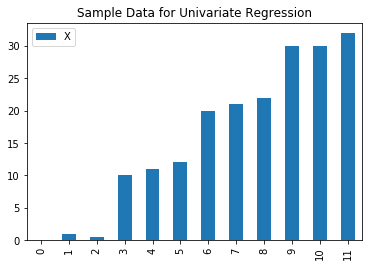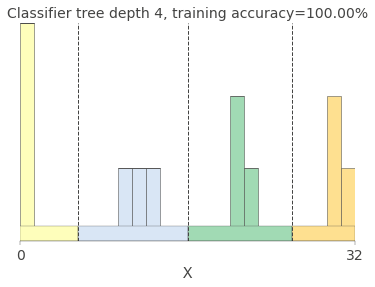# Split Up: dtreeviz (Part 5)

## Goal¶

This post aims to break down the module dtreeviz module step by step to fully understand what is implemented. After fully understanding this, I would like to contribute to this module and submit a pull request.

I really like this module and would like to see this works for other tree-based modules like XGBoost or Lightgbm. I found the exact same issue (issues 15) in github so I hope I could contribute to this issue.

This post is the 5th part:

• ctreeviz_univar

Reference

## trees.ctreeviz_univar¶

• L267: the beginning of the definition for ctreeviz_univar
• L272-275: treatment for pandas input
• L280-288: load decision tree classifier object as shadow_tree and other relevant attributes e.g., # of class, target values.
• L290-302: setting labels and spines visibility
• L304-319: plotting stacked bar chart with histogram when gtype=='barstacked'
• L320-330: plotting scatter plot with gitter
• L332: setting tick parameters
• L352-353: setting legend
• L355-358: setting a title
• L360-362: setting splits vertical line between categories
In :
from pathlib import Path
from graphviz.backend import run, view
import matplotlib.pyplot as plt
from numbers import Number
import matplotlib.patches as patches
import tempfile
import os
from sys import platform as PLATFORM
from colour import Color, rgb2hex
from typing import Mapping, List
from dtreeviz.utils import inline_svg_images, myround
from sklearn import tree
import graphviz

from dtreeviz.trees import *

# How many bins should we have based upon number of classes
NUM_BINS = [0, 0, 10, 9, 8, 6, 6, 6, 5, 5, 5]
# 0, 1, 2,  3, 4, 5, 6, 7, 8, 9, 10

def ctreeviz_univar(ax, x_train, y_train, max_depth, feature_name, class_names,
target_name,
fontsize=14, fontname="Arial", nbins=25, gtype='strip',
show={'title','legend','splits'},
colors=None):
if isinstance(x_train, pd.Series):
x_train = x_train.values
if isinstance(y_train, pd.Series):
y_train = y_train.values

#    ax.set_facecolor('#F9F9F9')
ct = tree.DecisionTreeClassifier(max_depth=max_depth)
ct.fit(x_train.reshape(-1, 1), y_train)

feature_names=[feature_name], class_names=class_names)

overall_feature_range = (np.min(x_train), np.max(x_train))
color_values = colors['classes'][n_classes]
color_map = {v: color_values[i] for i, v in enumerate(class_values)}
X_colors = [color_map[cl] for cl in class_values]

ax.set_xlabel(f"{feature_name}", fontsize=fontsize, fontname=fontname,
color=colors['axis_label'])
ax.spines['top'].set_visible(False)
ax.spines['right'].set_visible(False)
ax.yaxis.set_visible(False)
ax.spines['left'].set_visible(False)
ax.spines['bottom'].set_linewidth(.3)

X_hist = [x_train[y_train == cl] for cl in class_values]

if gtype == 'barstacked':
bins = np.linspace(start=overall_feature_range, stop=overall_feature_range, num=nbins, endpoint=True)
hist, bins, barcontainers = ax.hist(X_hist,
color=X_colors,
align='mid',
histtype='barstacked',
bins=bins,
label=class_names)

for patch in barcontainers:
for rect in patch.patches:
rect.set_linewidth(.5)
rect.set_edgecolor(colors['edge'])
ax.set_xlim(*overall_feature_range)
ax.set_xticks(overall_feature_range)
ax.set_yticks([0, max([max(h) for h in hist])])
elif gtype == 'strip':
# user should pass in short and wide fig
sigma = .013
mu = .08
class_step = .08
dot_w = 20
ax.set_ylim(0, mu + n_classes*class_step)
print('X_hist', X_hist)
for i, bucket in enumerate(X_hist):
y_noise = np.random.normal(mu+i*class_step, sigma, size=len(bucket))
ax.scatter(bucket, y_noise, alpha=.7, marker='o', s=dot_w, c=color_map[i],
edgecolors=colors['scatter_edge'], lw=.3)

ax.tick_params(axis='both', which='major', width=.3, labelcolor=colors['tick_label'],
labelsize=fontsize)

splits = [node.split() for node in shadow_tree.internal]
splits = sorted(splits)
bins = [ax.get_xlim()] + splits + [ax.get_xlim()]

pred_box_height = .07 * ax.get_ylim()
preds = []
for i in range(len(bins) - 1):
left = bins[i]
right = bins[i + 1]
inrange = y_train[(x_train >= left) & (x_train <= right)]
values, counts = np.unique(inrange, return_counts=True)
pred = values[np.argmax(counts)]
rect = patches.Rectangle((left, 0), (right - left), pred_box_height, linewidth=.3,
edgecolor=colors['edge'], facecolor=color_map[pred])
preds.append(pred)

if 'legend' in show:
add_classifier_legend(ax, class_names, class_values, color_map, target_name, colors)

if 'title' in show:
accur = ct.score(x_train.reshape(-1, 1), y_train)
title = f"Classifier tree depth {max_depth}, training accuracy={accur*100:.2f}%"
plt.title(title, fontsize=fontsize, color=colors['title'])

if 'splits' in show:
for split in splits:
plt.plot([split, split], [*ax.get_ylim()], '--', color=colors['split_line'], linewidth=1)


## Create a toy classification example¶

In :
import numpy as np
import graphviz
from sklearn import tree

X = np.array([0, 1, 0.5, 10, 11, 12, 20, 21, 22, 30, 30, 32]).reshape(-1, 1)
Y = np.array(['a', 'a', 'a', 'b', 'b', 'b', 'c', 'c', 'c', 'd', 'd', 'd']).reshape(-1, 1)
clf = tree.DecisionTreeClassifier(max_depth=3)
clf = clf.fit(X, Y)

df = pd.DataFrame(data={'X':X.ravel(), 'Y': Y.ravel()}, index=range(len(X)))
df.plot(kind='bar');
plt.title('Sample Data for Univariate Regression');## Visualize classification tree for univariate case¶

In :
fig, ax = plt.subplots(1)
ctreeviz_univar(ax, pd.Series(X.ravel()), pd.Series(Y.ravel()),
feature_name='X',
target_name='Y',
max_depth=4,
class_names=['a', 'b', 'c', 'd'],
gtype = 'barstacked',
show={'title', 'splits'}
)Note When I apply show={'legend'}, I obtained the error below and still not figured out yet what was wrong.

---------------------------------------------------------------------------
KeyError                                  Traceback (most recent call last)
<ipython-input-42-c31e8b14db34> in <module>
4                 target_name='Y',
5                 max_depth=4,
----> 6                 class_names=['a', 'b', 'c', 'd']
7                )

<ipython-input-41-b466a69d927c> in ctreeviz_univar(ax, x_train, y_train, max_depth, feature_name, class_names, target_name, fontsize, fontname, nbins, gtype, show, colors)
85         for i, bucket in enumerate(X_hist):
86             y_noise = np.random.normal(mu+i*class_step, sigma, size=len(bucket))
---> 87             ax.scatter(bucket, y_noise, alpha=.7, marker='o', s=dot_w, c=color_map[i],
88                        edgecolors=colors['scatter_edge'], lw=.3)
89

KeyError: 0